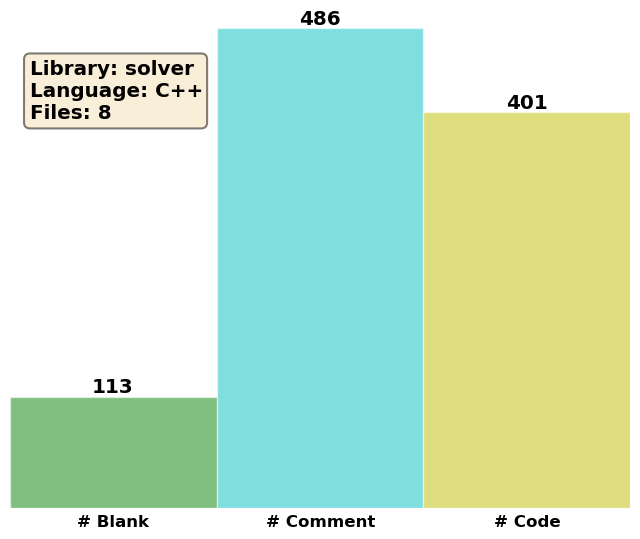# Solvers¶

We will here describe the inheritance hierarchy for generating solvers, in order to use and extend it properly. The runtime creation of solver objects relies on the Factory Method pattern [GHJV94][Ale01], implemented through the generic Factory class.## PCMSolver¶

class PCMSolver

Abstract Base Class for solvers inheritance hierarchy.

We use the Non-Virtual Interface idiom.

Author
Luca Frediani, Roberto Di Remigio
Date
2011, 2015

Subclassed by CPCMSolver, IEFSolver

Public Functions

void buildSystemMatrix(const Cavity &cavity, const IGreensFunction &gf_i, const IGreensFunction &gf_o)

Calculation of the PCM matrix.

Parameters
• cavity -

the cavity to be used

• gf_i -

Green’s function inside the cavity

• gf_o -

Green’s function outside the cavity

Eigen::VectorXd computeCharge(const Eigen::VectorXd &potential, int irrep = 0) const

Returns the ASC given the MEP and the desired irreducible representation.

Parameters
• potential -

the vector containing the MEP at cavity points

• irrep -

the irreducible representation of the MEP and ASC

Protected Functions

virtual void buildSystemMatrix_impl(const Cavity &cavity, const IGreensFunction &gf_i, const IGreensFunction &gf_o) = 0

Calculation of the PCM matrix.

Parameters
• cavity -

the cavity to be used

• gf_i -

Green’s function inside the cavity

• gf_o -

Green’s function outside the cavity

virtual Eigen::VectorXd computeCharge_impl(const Eigen::VectorXd &potential, int irrep = 0) const = 0

Returns the ASC given the MEP and the desired irreducible representation.

Parameters
• potential -

the vector containing the MEP at cavity points

• irrep -

the irreducible representation of the MEP and ASC

Protected Attributes

bool built_

Whether the system matrix has been built

bool isotropic_

Whether the solver is isotropic

## IEFSolver¶

class IEFSolver

IEFPCM, collocation-based solver.

Author
Luca Frediani, Roberto Di Remigio
Date
2011, 2015, 2016
Note
We store the non-Hermitian, symmetry-blocked T(epsilon) and Rinfinity matrices. The ASC is obtained by multiplying the MEP by Rinfinity and then using a partially pivoted LU decomposition of T(epsilon) on the resulting vector. In case the polarization weights are requested, we use the approach suggested in . First, the adjoint problem is solved:
$\mathbf{T}_\varepsilon^\dagger \tilde{v} = v$
Also in this case a partially pivoted LU decomposition is used. The “transposed” ASC is obtained by the matrix-vector multiplication:
$q^* = \mathbf{R}_\infty^\dagger \tilde{v}$
Eventually, the two sets of charges are summed and divided by 2 This avoids computing and storing the inverse explicitly, at the expense of storing both T(epsilon) and Rinfinity.

Inherits from PCMSolver

Public Functions

IEFSolver(bool symm)

Construct solver.

Parameters
• symm -

whether the system matrix has to be symmetrized

void buildAnisotropicMatrix(const Cavity &cavity, const IGreensFunction &gf_i, const IGreensFunction &gf_o)

Builds PCM matrix for an anisotropic environment.

Parameters
• cavity -

the cavity to be used.

• gf_i -

Green’s function inside the cavity

• gf_o -

Green’s function outside the cavity

void buildIsotropicMatrix(const Cavity &cavity, const IGreensFunction &gf_i, const IGreensFunction &gf_o)

Builds PCM matrix for an isotropic environment.

Parameters
• cavity -

the cavity to be used.

• gf_i -

Green’s function inside the cavity

• gf_o -

Green’s function outside the cavity

Private Functions

virtual void buildSystemMatrix_impl(const Cavity &cavity, const IGreensFunction &gf_i, const IGreensFunction &gf_o)

Calculation of the PCM matrix.

Parameters
• cavity -

the cavity to be used

• gf_i -

Green’s function inside the cavity

• gf_o -

Green’s function outside the cavity

virtual Eigen::VectorXd computeCharge_impl(const Eigen::VectorXd &potential, int irrep = 0) const

Returns the ASC given the MEP and the desired irreducible representation.

Parameters
• potential -

the vector containing the MEP at cavity points

• irrep -

the irreducible representation of the MEP and ASC

Private Members

bool hermitivitize_

Whether the system matrix has to be symmetrized

Eigen::MatrixXd Tepsilon_

T(epsilon) matrix, not symmetry blocked

std::vector<Eigen::MatrixXd> blockTepsilon_

T(epsilon) matrix, symmetry blocked form

Eigen::MatrixXd Rinfinity_

R_infinity matrix, not symmetry blocked

std::vector<Eigen::MatrixXd> blockRinfinity_

R_infinity matrix, symmetry blocked form

## CPCMSolver¶

class CPCMSolver

Solver for conductor-like approximation: C-PCM (COSMO)

Author
Roberto Di Remigio
Date
2013, 2016
Note
We store the scaled, Hermitian, symmetrized S matrix and use a robust Cholesky decomposition to solve for the ASC. This avoids computing and storing the inverse explicitly. The S matrix is already scaled by the dielectric factor entering the definition of the conductor model!

Inherits from PCMSolver

Public Functions

CPCMSolver(bool symm, double corr)

Construct solver.

Parameters
• symm -

whether the system matrix has to be symmetrized

• corr -

factor to correct the conductor results

Private Functions

virtual void buildSystemMatrix_impl(const Cavity &cavity, const IGreensFunction &gf_i, const IGreensFunction &gf_o)

Calculation of the PCM matrix.

Parameters
• cavity -

the cavity to be used

• gf_i -

Green’s function inside the cavity

• gf_o -

Green’s function outside the cavity

virtual Eigen::VectorXd computeCharge_impl(const Eigen::VectorXd &potential, int irrep = 0) const

Returns the ASC given the MEP and the desired irreducible representation.

Parameters
• potential -

the vector containing the MEP at cavity points

• irrep -

the irreducible representation of the MEP and ASC

Private Members

bool hermitivitize_

Whether the system matrix has to be symmetrized

double correction_

Correction for the conductor results

Eigen::MatrixXd S_

S matrix, not symmetry blocked

std::vector<Eigen::MatrixXd> blockS_

S matrix, symmetry blocked form